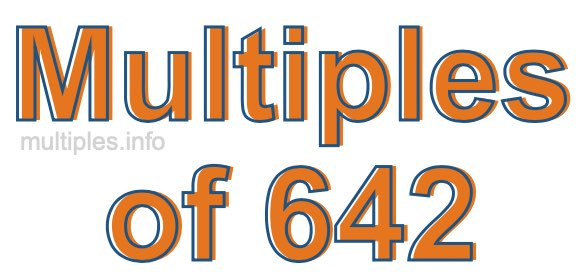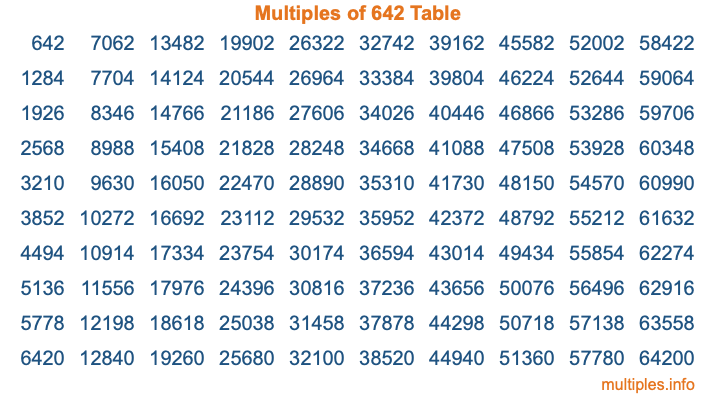Multiples of 642Welcome to the Multiples of 642 page. Here we will first teach you everything you will ever need to know about the multiples of 642, and then give you a study guide summary of everything we taught you to make sure you remember it all. Use this page to look up facts and learn information about the multiples of 642. This page will make you a multiples of six hundred forty-two expert!

Definition of Multiples of 642
Multiples of 642 are all the numbers that when divided by 642 equal an integer. Each of the multiples of 642 are called a multiple. A multiple of 642 is created by multiplying 642 by an integer.

Therefore, to create a list of multiples of 642, you start with 1 multiplied by 642, then 2 multiplied by 642, then 3 multiplied by 642, and so on for as long as you want. Thus, the list of the first five multiples of 642 is 642, 1284, 1926, 2568, and 3210. To see a larger list of multiples of 642, see the printable image of Multiples of 642 further down on this page. We also have a category where you can choose any nth multiple of 642.

Multiples of 642 Checker
The Multiples of 642 Checker below checks to see if any number of your choice is a multiple of 642. In other words, it checks to see if there is any number (integer) that when multiplied by 642 will equal your number. To do that, we divide your number by 642. If the the quotient is an integer, then your number is a multiple of 642.

Is  a multiple of 642?

Least Common Multiple of 642 and ...
A Least Common Multiple (LCM) is the lowest multiple that two or more numbers have in common. This is also called the smallest common multiple or lowest common multiple and is useful to know when you are adding our subtracting fractions. Enter one or more numbers below (642 is already entered) to find the LCM.

Check out our LCM Calculator if you need more details about the Least Common Multiple or if you need the LCM for different numbers for adding and subtraction fractions.

nth Multiple of 642
As we stated above, 642 is the first multiple of 642, 1284 is the second multiple of 642, 1926 is the third multiple of 642, and so on. Enter a number below to find the nth multiple of 642.

th multiple of 642

Multiples of 642 vs Factors of 642
642 is a multiple of 642 and a factor of 642, but that is where the similarities end. All postive multiples of 642 are 642 or greater than 642. All positive factors of 642 are 642 or less than 642.

Below is the beginning list of multiples of 642 and the factors of 642 so you can compare:

Multiples of 642: 642, 1284, 1926, 2568, 3210, etc.

Factors of 642: 1, 2, 3, 6, 107, 214, 321, 642

As you can see, the multiples of 642 are all the numbers that you can divide by 642 to get a whole number. The factors of 642, on the other hand, are all the whole numbers that you can multiply by another whole number to get 642.

It's also interesting to note that if a number (x) is a factor of 642, then 642 will also be a multiple of that number (x).

Multiples of 642 vs Divisors of 642
The divisors of 642 are all the integers that 642 can be divided by evenly. Below is a list of the divisors of 642.

Divisors of 642: 1, 2, 3, 6, 107, 214, 321, 642

The interesting thing to note here is that if you take any multiple of 642 and divide it by a divisor of 642, you will see that the quotient is an integer.

Multiples of 642 Table
Below is an image of the first 100 multiples of 642 in a table. The table is in chronological order, column by column. The first column has the first ten multiples of 642, the second column has the next ten multiples of 642, and so on.The Multiples of 642 Table is also referred to as the 642 Times Table or Times Table of 642. You are welcome to print out our table for your studies.

Negative Multiples of 642
Although not often discussed or needed in math, it is worth mentioning that you can make a list of negative multiples of 642 by multiplying 642 by -1, then by -2, then by -3, and so on, to get the following list of negative multiples of 642:

-642, -1284, -1926, -2568, -3210, etc.

Multiples of 642 Summary
Below is a summary of important Multiples of 642 facts that we have discussed on this page. To retain the knowledge on this page, we recommend that you read through the summary and explain to yourself or a study partner why they hold true.

There are an infinite number of multiples of 642.

A multiple of 642 divided by 642 will equal a whole number.

642 divided by a factor of 642 equals a divisor of 642.

The nth multiple of 642 is n times 642.

The largest factor of 642 is equal to the first positive multiple of 642.

642 is a multiple of every factor of 642.

642 is a multiple of 642.

A multiple of 642 divided by a divisor of 642 equals an integer.

642 divided by a divisor of 642 equals a factor of 642.

Any integer times 642 will equal a multiple of 642.

Multiples of a Number
Here you can get the multiples of another number, all with the same attention to detail as we did for multiples of 642 on this page.

Multiples of
Multiples of 643
Did you find our page about multiples of six hundred forty-two educational? Do you want more knowledge? Check out the multiples of the next number on our list!